# C++多态的实现原理剖析

## 1、虚函数表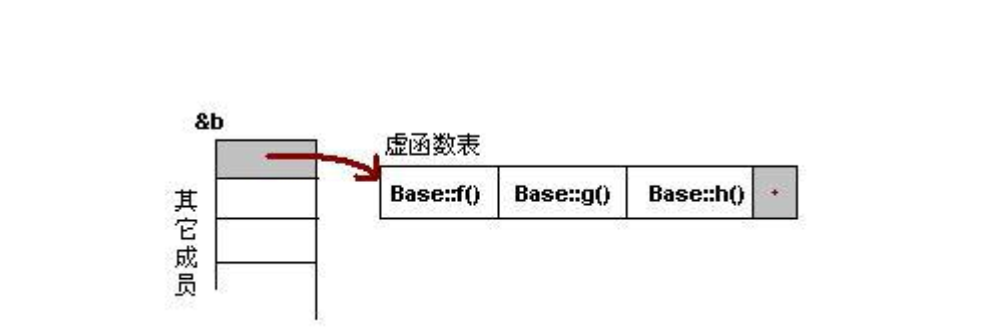``````class Base
{
public:
virtual void f() { cout << "Base::f" << endl; }
virtual void g() { cout << "Base::g" << endl; }
virtual void h() { cout << "Base::h" << endl; }
};

typedef void (*FUNC)();

int main()
{
cout<<"Base size:"<<sizeof(Base)<<endl;
Base b;
cout<<"对象的起始地址： "<<&b<<endl;
//这是在取对象的地址，取出来的就是对象的首地址
//对象的起始地址，就类似一个函数指针数组的指针(就是虚函数表的指针)

cout<<"虚函数表的地址： "<<(int**)*(int *)&b<<endl;
//在取出起始地址&b之后，我们只想取得前四个字节，所以将上面的地址强制类型转为int*型，再解引用，就是虚函数表的地址，虚函数表的起始地址，再进行强制类型转化成int**

cout<<"虚函数表第一个函数的地址:"<<*((int**)*(int *)&b)<<endl;
//这个就比上面的多了一个*，就是解引用，对虚函数表首地址解引用，得到虚函数表的第一个函数的地址

cout<<"虚函数表第二个函数的地址:"<<*((int**)*(int *)&b+1)<<endl;
//这个比上边的多了一个+1，可以看出是指针加1，所以地址实际+4，指向下一个函数

//注意不要转为 FUNC 来打印， cout 没有重载
FUNC pf = (FUNC)(*((char**)*(int *)&b));
pf();
pf = (FUNC)(*((void**)*(int *)&b+1));
pf();
pf = (FUNC)(*((void**)*(int *)&b+2));
pf();
return 0;
}``````

## 2、一般继承（无虚函数覆写）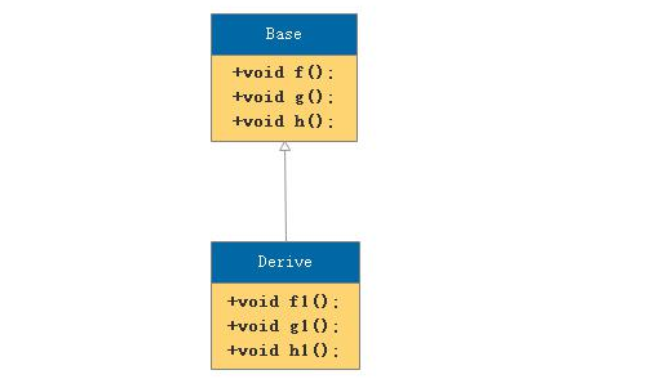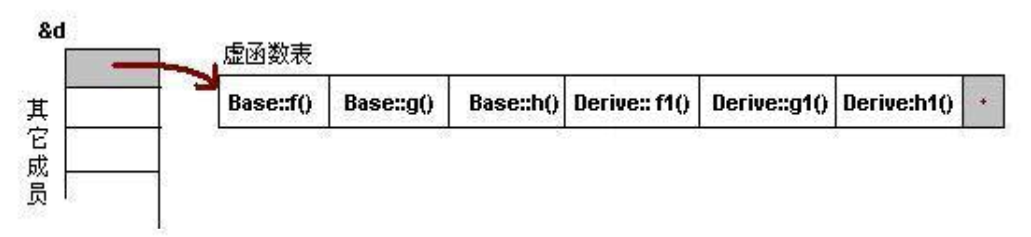## 3、一般继承(有虚函数覆写)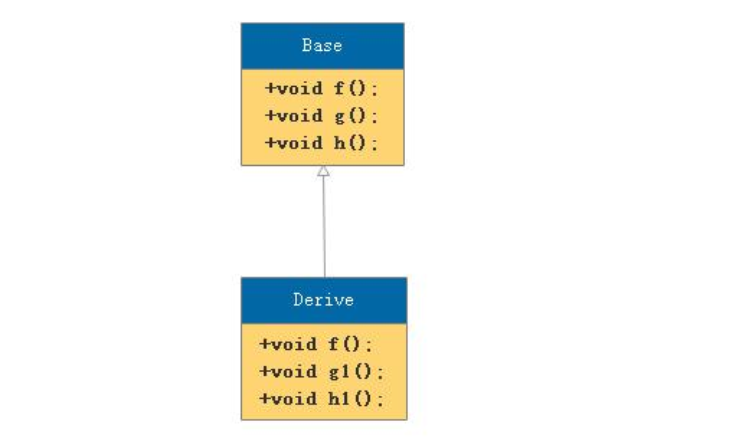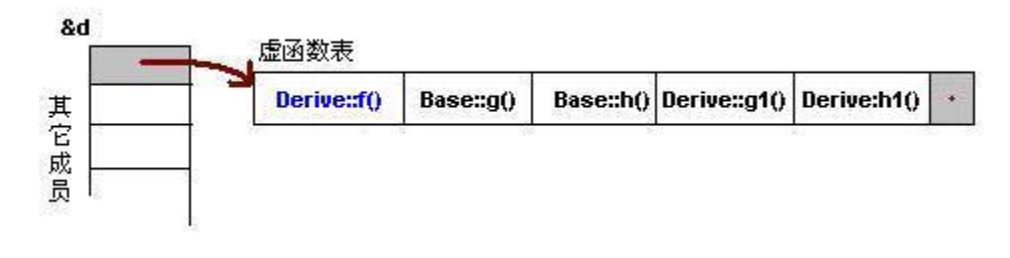``````Base *b = new Derive();
b->f();``````

``````Base *b = new Derive();
b->f();``````

1， 明确 b 类型。
2， 然后通过指针虚函数表的指针 vptr 和偏移量,匹配虚函数的入口。
3， 根据入口地址调用虚函数。

Last modification：August 7th, 2019 at 09:01 pm